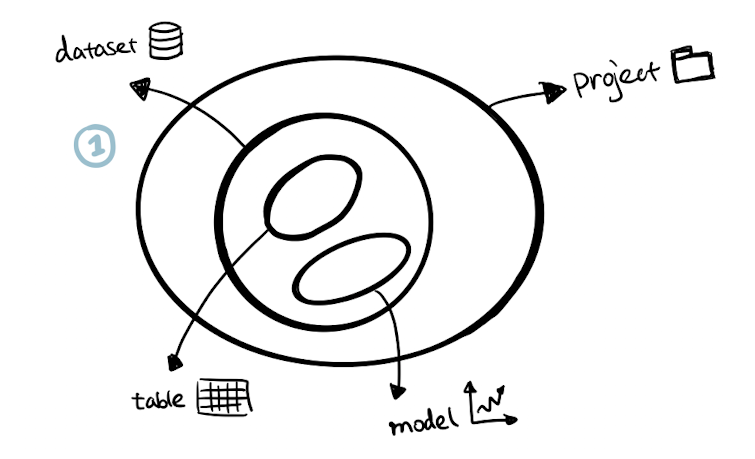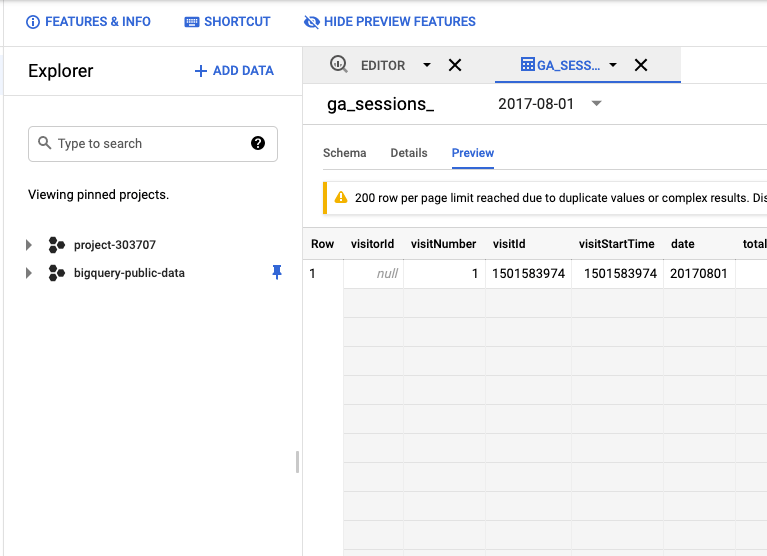top of pageSearch

# How to Build ML Model using BigQueryWhile taking the first step into the field of machine learning, it is so easy to get overwhelmed by all sorts of complex algorithms and ugly symbols. Therefore, hopefully, this article can lower the entry barrier by providing a beginner-friendly guide. Allow you to get a sense of achievement by building your own ML model using BigQuery and SQL. That's right, we can use SQL to implement machine learning. If you are looking for several lines of code to get your hands dirty in the ML field, please continue reading :)

## 1. Set Up the Basic Structure 📁In a nutshell, BigQuery project contains datasets and a dataset contains tables and models.

1) Navigate to BigQuery in the left sidebar of the Google Cloud Platform Console and then create a project in Google Cloud Platform, name it as anything you want (e.g. “project” in this example)2) With the "SQL Workspace" panel, select your project and then click “Create Dataset” and give your dataset a name (e.g. analytics).3) Click “Add data" and select "Explore public data sets". From the public dataset library, search google analytics sample and add it to your project. Have a read of the Google Analytics sample schema if interested.4) Explore the dataset by navigating to the "ga_sessions_" in the "bigquery-public-data" and select the "Preview" tab. It is a massive dataset with multiple columns and a collection of subsets which are partitioned by date (e.g. "2017-08-01" in the example below). Notice that the dataset sits within the project "bigquery-public-data" rather than our own project "project-303707", but we can still query this dataset from our project as it is publicly accessible.## 2. What's Your Problem 🤨 ?Before jumping into building the model, clarify the problem we are trying to solve by asking the following questions:

1) What is the label?

A label is a variable to be predicted. In this example, I will predict whether the website visitor will make any transactions and I gave this label the name "purchase". This can be derived from the existing variable "totals.transactions".

2) How is the label value defined?

For simplicity, let's make this prediction a black or white situation, either "purchase" or "no purchase". Since the model training cannot handle string value as the output result, therefore it is necessary to code them into numbers.

• purchase = 1: the visitor will make transactions → "totals.transactions > 0" is true

• purchase = 0: the visitor won't make any transactions → "totals.transactions > 0" is false

This criterion can be defined using an IF statement in SQL:

`IF(totals.transactions > 0, 1, 0) as purchase`

3) What are the features/predictors?

Predictors are the features selected to predict the label. For this simple model, "totals.pageviews", "device.isMobile", "totals.timeonsite" are selected as the predictors. Let's find out if they are actually good predictors to estimate the chance of making purchase.

4) What do we already know? What are we trying to predict?

We used the data that we already know to build the model. For this exercise, I chose a range of subsets with date between 2017-01-01 and 2017-07-01 from the google analytics dataset. We have already known if these records have made the purchase or not based on the "totals.transactions" value.

I chose the subsets from 2017-07-01 to 2017-08-01 as the prediction set. Although we already know if they have made any transactions from the data. But for illustration purposes, let's assume that we only know the predictors and use them to predict the label.

5) What type of model should we choose?

There are two major types of problems in machine learning: classification and regression. Classification problem is to predict categorical data (e.g. true/false, cat/dog) whereas regression problem is to numerical data with continuous quantity (e.g. temperature, revenue etc). Since the label "purchase" is designed as a category with discrete value (either 1 or 0), this is a classification problem. Logistic regression, decision tree, support vector machine are commonly used models to solve classification. I choose logistic regression because it is the easiest to start with and also it is supported by BigQuery ML.

### 3. Build the Model 📊Building ML models in BigQuery split into training dataset and evaluation dataset.

• Training dataset: a subset of sample data used to create the model.

• Evaluation dataset: a subset of sample data used to assess the performance of the model.

In this example, data subset with date from "2017-01-01" to "2017-05-01" is used for training and "2017-05-01" to "2017-07-01" is used for evaluation.

1) Train the Model

```CREATE OR REPLACE MODEL `analytics.my_model`
OPTIONS
(
model_type = "logistic_reg",
labels = ['purchase']
)
AS

SELECT *
EXCEPT(fullvisitorid)
FROM
(SELECT fullvisitorid,
IFNULL(totals.pageviews, 0) AS page,
IFNULL(totals.timeonsite, 0) AS duration,
CASE
WHEN device.isMobile is True THEN 1
ELSE 0
END mobile
WHERE _TABLE_SUFFIX BETWEEN "20170101" AND "20170501"
)

JOIN
(SELECT fullvisitorid,
IF(totals.transactions > 0, 1, 0) as purchase
) target
USING (fullvisitorid)

```

Let’s break down this chunk of code into pieces:

```CREATE OR REPLACE MODEL `analytics.my_model`
OPTIONS
(
model_type = "logistic_reg",
labels = ['purchase']
)
AS

```

The first part creates the model "my_model" within the "analytics" dataset by passing two parameters to the OPTIONS clause:

• Model type: “logistic_reg” - logistic regression model

• Labels: “purchase” - the variable we are predicting

The second part, after the “AS” keyword, specifies the training dataset:

```SELECT *
EXCEPT(fullvisitorid)
FROM
(SELECT fullvisitorid,
IFNULL(totals.pageviews, 0) AS page,
IFNULL(totals.timeonsite, 0) AS duration,
CASE
WHEN device.isMobile is True THEN 1
ELSE 0
END mobile
WHERE _TABLE_SUFFIX BETWEEN "20170101" AND "20170501"
)
JOIN
(SELECT fullvisitorid,
IF(totals.transactions > 0, 1, 0) as purchase
) target
USING (fullvisitorid)

```

We are joining two tables together over here, the first one select all features which are used as predictors in this scenario:

• "totals.pageviews": indicates the number of page views within the session and then apply IFNULL function to replace the null value with 0

• "totals.timeonsite": indicates the time spent within the session and then apply IFNULL function to replace the null value with 0

• "device.isMobile": indicates if the visitor uses a mobile device and then apply CASE function to transform boolean (true or false) into numeric values (1 or 0)

The keyword "_TABLE_SUFFIX" is used to filter the dataset so that only the training subset of "ga_session" is included (2017-01-01" to "2017-05-01").

Then we join the first table containing the predictors with the second table that includes each visitor's "purchase" label defined by the statement "IF(totals.transactions > 0, 1, 0)".

Then we exclude the fullvisitorid from the joined table using the statement "SELECT * EXCEPT(fullvisitorid) FROM ..." because ID doesn't contribute to the prediction of purchase.

After running the training statement in the query composer panel, we will be able to see "my_model" appeared in the "analytics" dataset.2) Evaluate the Model

```SELECT
roc_auc
FROM ML.EVALUATE(MODEL analytics.my_model,

(
SELECT *
EXCEPT(fullvisitorid)
FROM
(SELECT fullvisitorid,
IFNULL(totals.pageviews, 0) AS page,
IFNULL(totals.timeonsite, 0) AS duration,
CASE
WHEN device.isMobile is True THEN 1
ELSE 0
END mobile
WHERE _TABLE_SUFFIX BETWEEN "20170501" AND "20170701"
)

JOIN
(SELECT fullvisitorid,
IF(totals.transactions > 0, 1, 0) as purchase
) target
USING (fullvisitorid)
));

```

This can be broken down into two layers.

```-- outer layer
SELECT roc_auc
FROM ML.EVALUATE(...)

```

The outer layer is to select the metric from the result returned from the ML.EVALUATE clause. In this case, only "roc_auc" is selected, but we can also use "SELECT *" to get other common metrics to evaluate classification models such as precision, recall, accuracy, f1_score and log_loss.

```-- inner layer
MODEL analytics.my_model,
(
SELECT *
EXCEPT(fullvisitorid)
FROM
(SELECT fullvisitorid,
IFNULL(totals.pageviews, 0) AS page,
IFNULL(totals.timeonsite, 0) AS duration,
CASE
WHEN device.isMobile is True THEN 1
ELSE 0
END mobile
WHERE _TABLE_SUFFIX BETWEEN "20170501" AND "20170701"
)

JOIN
(SELECT fullvisitorid,
IF(totals.transactions > 0, 1, 0) as purchase
) target
USING (fullvisitorid)
)

```

The inner layer is to pass the model "analytics.my_model" and the evaluation dataset as the parameters of this EVALUATE function. As we can see, the evaluation dataset is almost identical to the definition of the training dataset, except that the _TABLE_SUFFIX has been changed into another set of data range between "20170501" and "2017070.The code returned the roc_auc value as 0.75. With this value, we can only say that this is a decent but not good enough model. There are many ways to improve its performance, for instance, dive deeper into feature selection by introducing more informative features as the predictors. I encourage you to have a think about it, what other attributes may contribute to the prediction of "purchase". Give it a go to implement it and reach a higher roc_auc score. Think of it as an iterative process that continuously tests the performance of the model and improves on it, until it is reaching an optimal value (e.g. roc_auc > 0.9).

Feel free to comment down below if you thought of any approaches to improve the score.

## 4. Make Prediction 📈Comparing the prediction statement below to the training or evaluation statement, there is apparent difference that only predictors (page, duration and mobile) are selected. This is because the label will be the query output rather than the input.

```SELECT
*
FROM
ML.PREDICT(MODEL `analytics.my_model`,
(
SELECT fullvisitorid,
IFNULL(totals.pageviews, 0) AS page,
IFNULL(totals.timeonsite, 0) AS duration,
CASE
WHEN device.isMobile is True THEN 1
ELSE 0
END mobile
WHERE _TABLE_SUFFIX BETWEEN "20170701" AND "20170801"
)
);

```

For each predictive model in BigQuery, the predicted label will be named as "predicted_<label_column_name>", e.g. predicted_purchase. Additionally, we are choosing a new set of data "2017-07-01" to "2017-08-01" which is outside the range of original dataset used for training and evaluation. Ideally, it is the dataset that we only have information of the features but we don't know if they have made any purchases.

The result below shows the predicted label of each visitor as well as the probability of being labeled as 1 or 0 respectively.## Take-Home Message

This article briefly introduces how to build a simple machine learning model using SQL in BigQuery, with four major steps being covered:

1. Set up the basic structure

2. Be clear about the problem

3. Build the model (train and evaluate the model)

4. Make the prediction

Hopefully, it helps to ease the friction of entering the machine learning field. Most importantly, let's learn and grow together!